# Example of the Euler Method in 2D

We will use the Euler method to solve the damped pendulum equation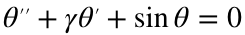which we first rewrite as a systemfor initial conditions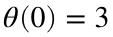,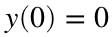, and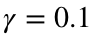.
Set up initial conditions and parameters
theta0=3;
y0=0;
gamma=0.1;
Set up time stepping
h = 1/100;
tFinal=20;
nSteps=tFinal/h;
t=linspace(0,tFinal,nSteps+1);
Initialize θ & y
theta=zeros(size(t));
y = zeros(size(t));
theta(1)=theta0; y(1)=y0;
Loop through and run the Euler method. Note that this code uses a function (defined at the bottom of this file) to evaluate the right-hand side.
for k=1:nSteps
[delta_x, delta_y] = pendulumODE(theta(k),y(k),gamma);
theta(k+1) = theta(k) + h * delta_x;
y(k+1) = y(k) + h * delta_y;
end
Plot the results
plot(t,theta,t,y);
xlabel('t'); legend('\theta','y')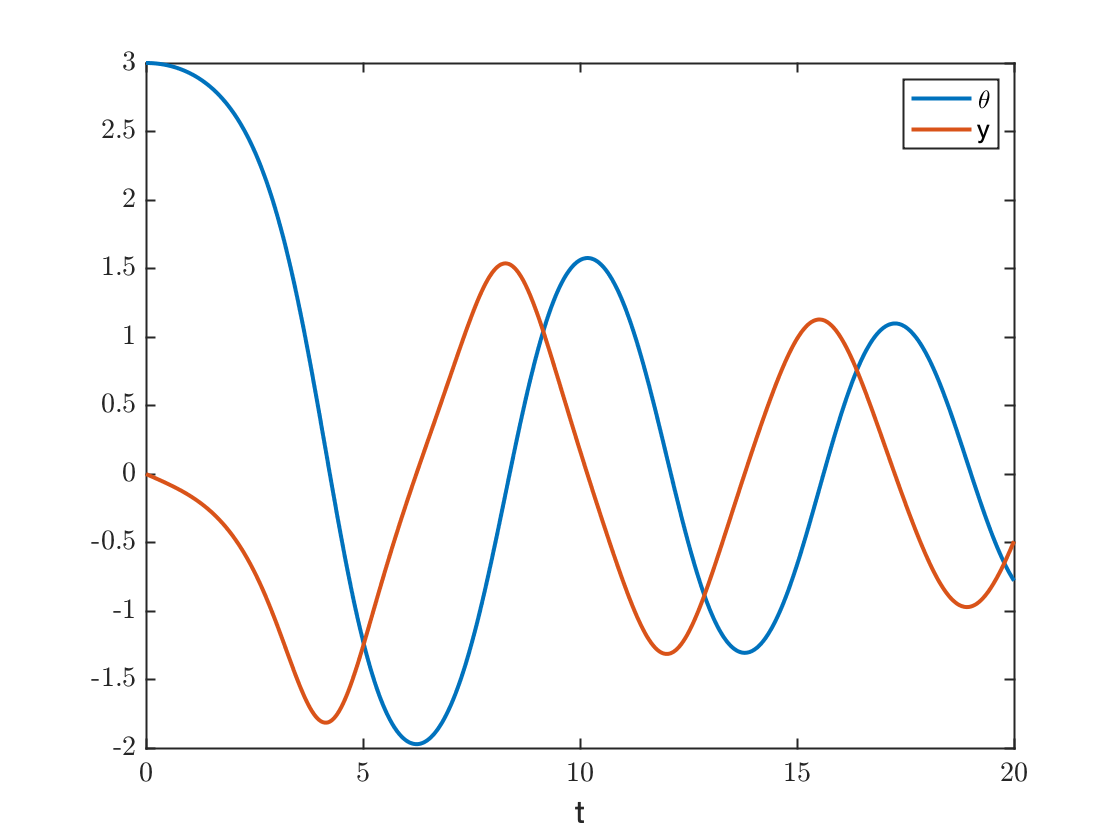Plot the solution in the phase plane
plot(theta,y)
xlabel('\theta');ylabel('y');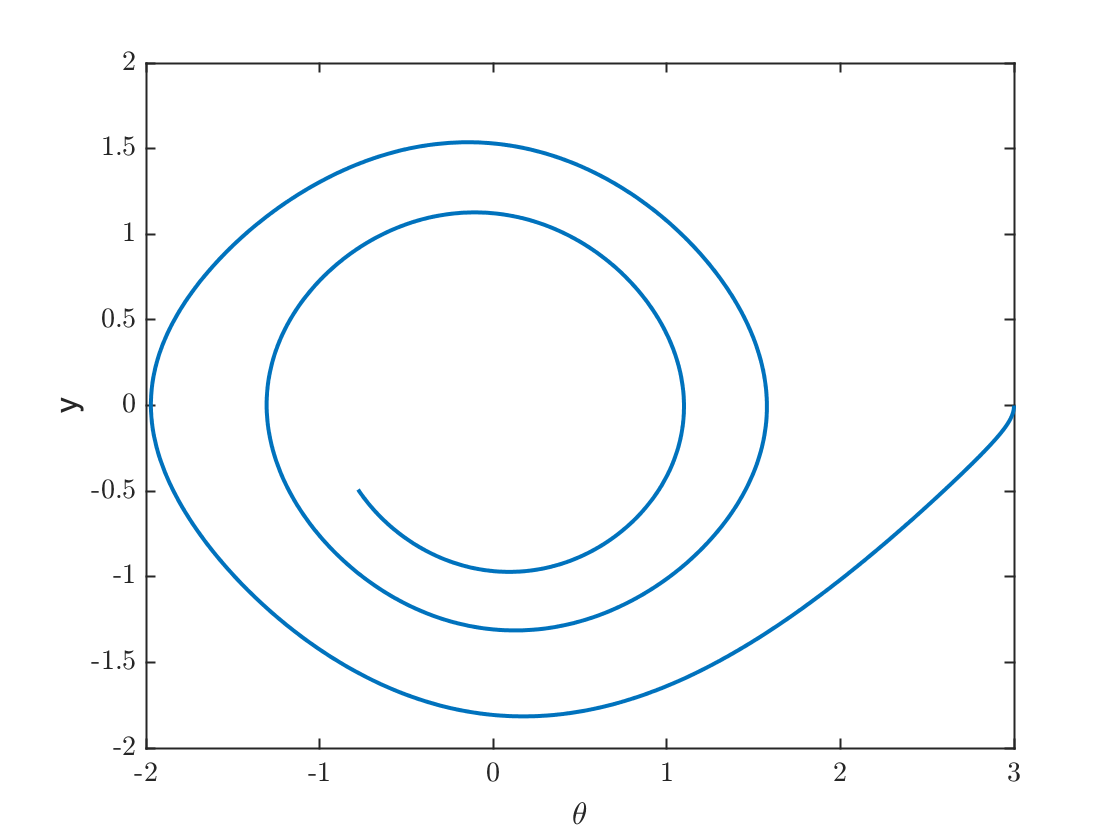#### Function used to evaluate the differential equation

function [delta_x, delta_y] = pendulumODE(x,y,gamma)
delta_x = y;
delta_y = -gamma*y-sin(x);
end